# matplotlib.pyplot.gca¶

matplotlib.pyplot.gca(**kwargs)[source]

Get the current Axes instance on the current figure matching the given keyword args, or create one.

matplotlib.figure.Figure.gca
The figure's gca method.

Examples

To get the current polar axes on the current figure:

plt.gca(projection='polar')


If the current axes doesn't exist, or isn't a polar one, the appropriate axes will be created and then returned.

## Examples using matplotlib.pyplot.gca¶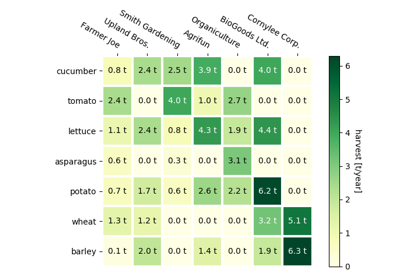Creating annotated heatmaps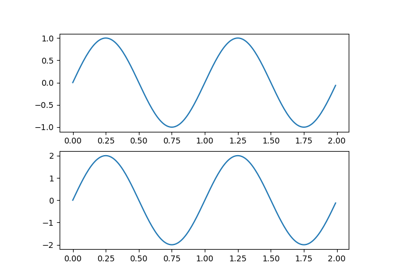Multiple Figs Demo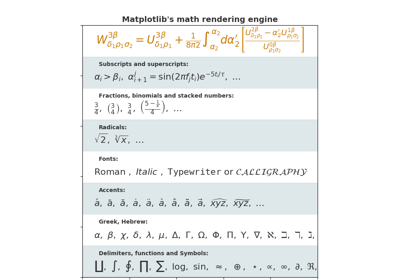Mathtext Examples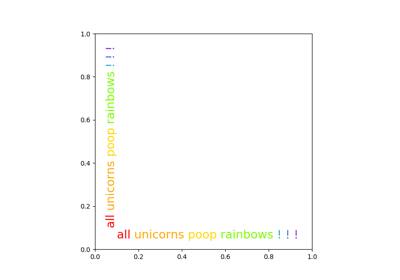Rainbow text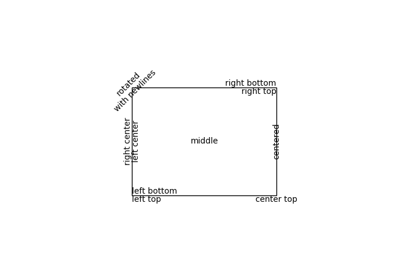Precise text layout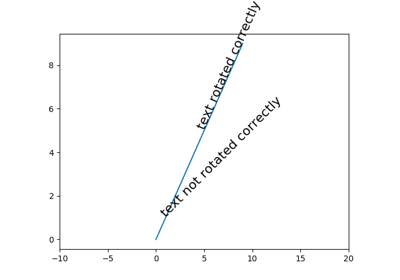Text Rotation Relative To Line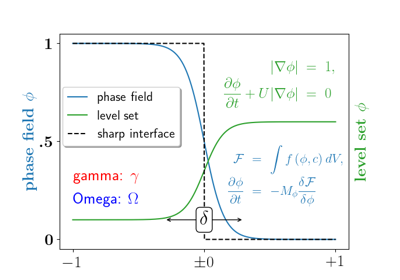Usetex Demo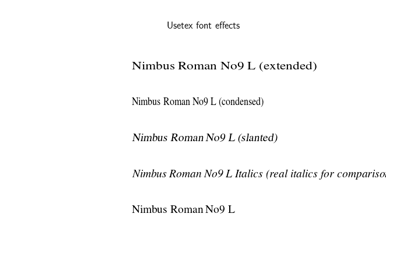Usetex Fonteffects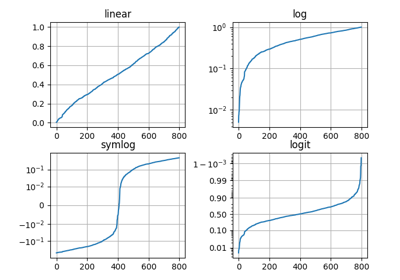Pyplot Scales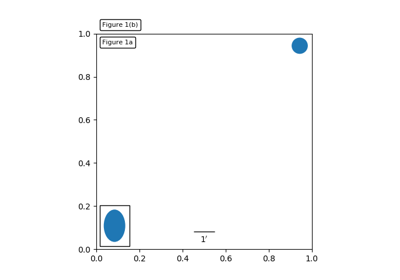Simple Anchored ArtistsInteractive functions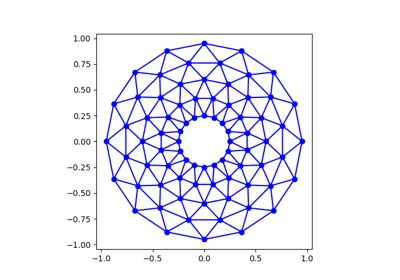Trifinder Event Demo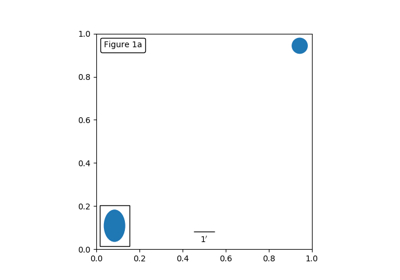Anchored Artists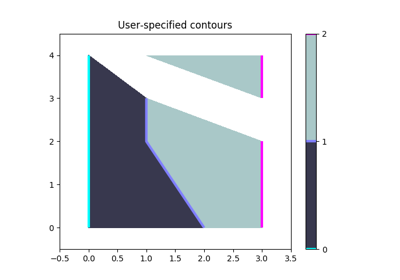Manual Contour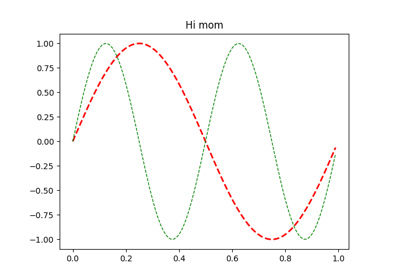Set And Get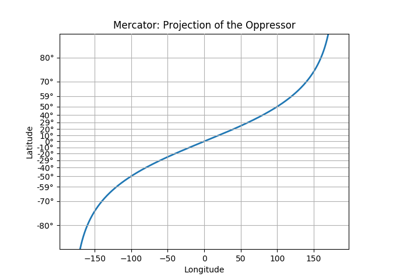Custom scale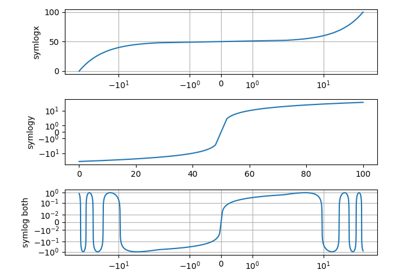Symlog Demo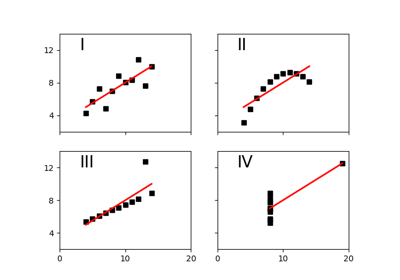Anscombe's Quartet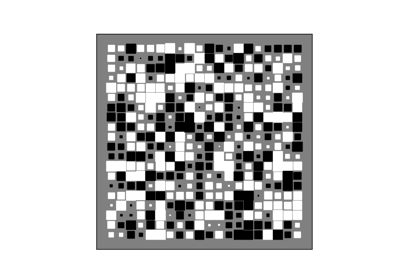Hinton diagrams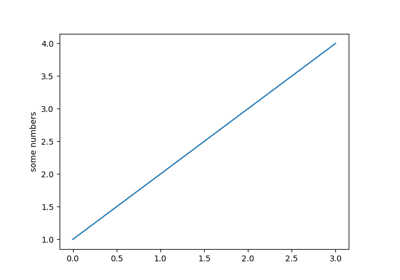Pyplot tutorial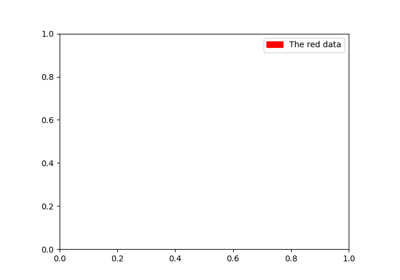Legend guide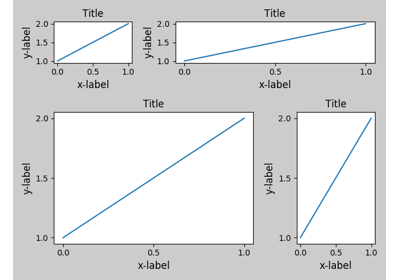Tight Layout guide